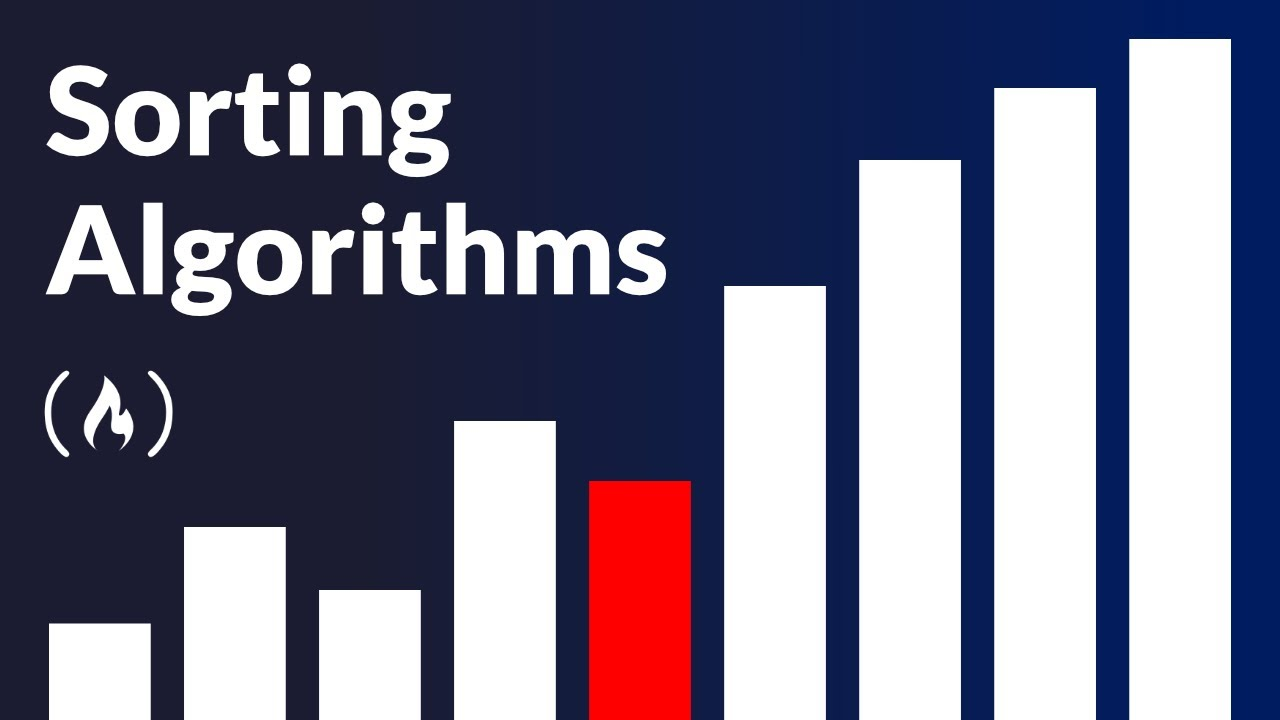# Understanding Sorting AlgorithmsLearn some of the most popular sorting algorithms! You will learn selection sort, bubble sort, insertion sort, merge sort, and their order of complexities! This video uses C++ but the concepts apply to any programming language.

Learn some of the most popular sorting algorithms! You will learn selection sort, bubble sort, insertion sort, merge sort, and their order of complexities! This video uses C++ but the concepts apply to any programming language.

💻 Source code on GitHub: https://github.com/codingcleverly/sorting_algorithms_freecodecamp

⭐️ Course Contents ⭐️
⌨️ (0:00:00) Introduction
⌨️ (0:01:06) Simple Sorting Algorithm
⌨️ (0:16:16) Selection Sort
⌨️ (0:25:56) Diagrammatic Explanation
⌨️ (0:28:40) Bubble Sort
⌨️ (0:35:20) Graphical Explanation of BubbleSort
⌨️ (0:38:51) Insertion Sort
⌨️ (0:45:08) Graphical Implementation
⌨️ (0:49:30) Merge Sort
⌨️ (0:51:57) Extensive Explanation
⌨️ (1:09:37) Difference b/w the Algorithms
⌨️ (1:10:46) Conclusion